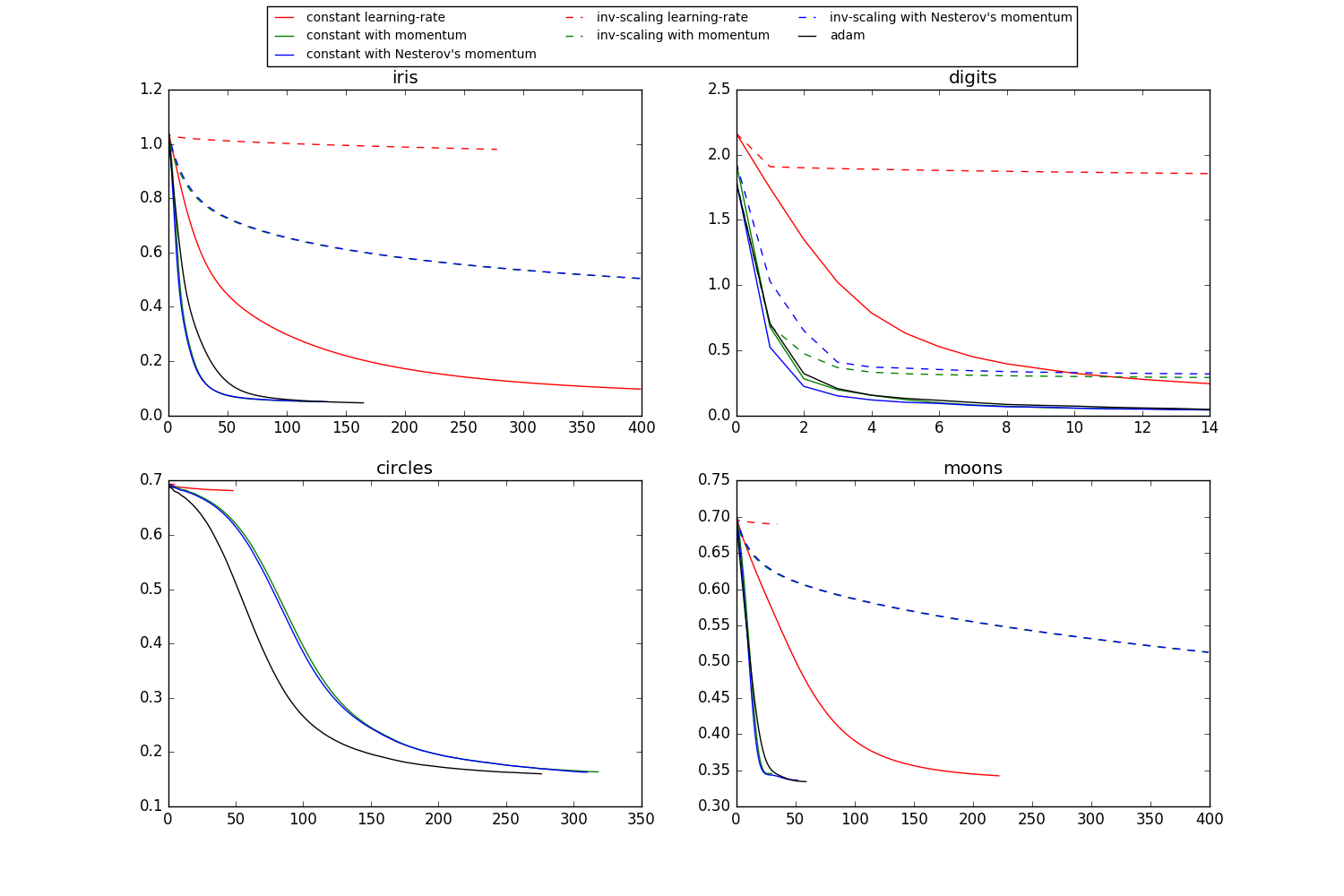# Compare Stochastic learning strategies for MLPClassifier¶

This example visualizes some training loss curves for different stochastic learning strategies, including SGD and Adam. Because of time-constraints, we use several small datasets, for which L-BFGS might be more suitable. The general trend shown in these examples seems to carry over to larger datasets, however.

Note that those results can be highly dependent on the value of `learning_rate_init`.Out:

```  learning on dataset iris
training: constant learning-rate
Training set score: 0.980000
Training set loss: 0.096922
training: constant with momentum
Training set score: 0.980000
Training set loss: 0.050260
training: constant with Nesterov's momentum
Training set score: 0.980000
Training set loss: 0.050277
training: inv-scaling learning-rate
Training set score: 0.360000
Training set loss: 0.979983
training: inv-scaling with momentum
Training set score: 0.860000
Training set loss: 0.504017
training: inv-scaling with Nesterov's momentum
Training set score: 0.860000
Training set loss: 0.504760
Training set score: 0.980000
Training set loss: 0.046248

learning on dataset digits
training: constant learning-rate
Training set score: 0.956038
Training set loss: 0.243802
training: constant with momentum
Training set score: 0.992766
Training set loss: 0.041297
training: constant with Nesterov's momentum
Training set score: 0.993879
Training set loss: 0.042898
training: inv-scaling learning-rate
Training set score: 0.638843
Training set loss: 1.855465
training: inv-scaling with momentum
Training set score: 0.912632
Training set loss: 0.290584
training: inv-scaling with Nesterov's momentum
Training set score: 0.909293
Training set loss: 0.318387
Training set score: 0.991653
Training set loss: 0.045934

learning on dataset circles
training: constant learning-rate
Training set score: 0.830000
Training set loss: 0.681498
training: constant with momentum
Training set score: 0.940000
Training set loss: 0.163712
training: constant with Nesterov's momentum
Training set score: 0.940000
Training set loss: 0.163012
training: inv-scaling learning-rate
Training set score: 0.500000
Training set loss: 0.692855
training: inv-scaling with momentum
Training set score: 0.510000
Training set loss: 0.688376
training: inv-scaling with Nesterov's momentum
Training set score: 0.500000
Training set loss: 0.688593
Training set score: 0.930000
Training set loss: 0.159988

learning on dataset moons
training: constant learning-rate
Training set score: 0.850000
Training set loss: 0.342245
training: constant with momentum
Training set score: 0.850000
Training set loss: 0.345580
training: constant with Nesterov's momentum
Training set score: 0.850000
Training set loss: 0.336284
training: inv-scaling learning-rate
Training set score: 0.500000
Training set loss: 0.689729
training: inv-scaling with momentum
Training set score: 0.830000
Training set loss: 0.512595
training: inv-scaling with Nesterov's momentum
Training set score: 0.830000
Training set loss: 0.513034
Training set score: 0.850000
Training set loss: 0.334243
```

```print(__doc__)
import matplotlib.pyplot as plt
from sklearn.neural_network import MLPClassifier
from sklearn.preprocessing import MinMaxScaler
from sklearn import datasets

# different learning rate schedules and momentum parameters
params = [{'solver': 'sgd', 'learning_rate': 'constant', 'momentum': 0,
'learning_rate_init': 0.2},
{'solver': 'sgd', 'learning_rate': 'constant', 'momentum': .9,
'nesterovs_momentum': False, 'learning_rate_init': 0.2},
{'solver': 'sgd', 'learning_rate': 'constant', 'momentum': .9,
'nesterovs_momentum': True, 'learning_rate_init': 0.2},
{'solver': 'sgd', 'learning_rate': 'invscaling', 'momentum': 0,
'learning_rate_init': 0.2},
{'solver': 'sgd', 'learning_rate': 'invscaling', 'momentum': .9,
'nesterovs_momentum': True, 'learning_rate_init': 0.2},
{'solver': 'sgd', 'learning_rate': 'invscaling', 'momentum': .9,
'nesterovs_momentum': False, 'learning_rate_init': 0.2},

labels = ["constant learning-rate", "constant with momentum",
"constant with Nesterov's momentum",
"inv-scaling learning-rate", "inv-scaling with momentum",

plot_args = [{'c': 'red', 'linestyle': '-'},
{'c': 'green', 'linestyle': '-'},
{'c': 'blue', 'linestyle': '-'},
{'c': 'red', 'linestyle': '--'},
{'c': 'green', 'linestyle': '--'},
{'c': 'blue', 'linestyle': '--'},
{'c': 'black', 'linestyle': '-'}]

def plot_on_dataset(X, y, ax, name):
# for each dataset, plot learning for each learning strategy
print("\nlearning on dataset %s" % name)
ax.set_title(name)
X = MinMaxScaler().fit_transform(X)
mlps = []
if name == "digits":
# digits is larger but converges fairly quickly
max_iter = 15
else:
max_iter = 400

for label, param in zip(labels, params):
print("training: %s" % label)
mlp = MLPClassifier(verbose=0, random_state=0,
max_iter=max_iter, **param)
mlp.fit(X, y)
mlps.append(mlp)
print("Training set score: %f" % mlp.score(X, y))
print("Training set loss: %f" % mlp.loss_)
for mlp, label, args in zip(mlps, labels, plot_args):
ax.plot(mlp.loss_curve_, label=label, **args)

fig, axes = plt.subplots(2, 2, figsize=(15, 10))
# load / generate some toy datasets
data_sets = [(iris.data, iris.target),
(digits.data, digits.target),
datasets.make_circles(noise=0.2, factor=0.5, random_state=1),
datasets.make_moons(noise=0.3, random_state=0)]

for ax, data, name in zip(axes.ravel(), data_sets, ['iris', 'digits',
'circles', 'moons']):
plot_on_dataset(*data, ax=ax, name=name)

fig.legend(ax.get_lines(), labels=labels, ncol=3, loc="upper center")
plt.show()
```

Total running time of the script: (0 minutes 5.879 seconds)

Download Python source code: `plot_mlp_training_curves.py`